# What is 1D 2D and 3D

## Comparison between 1D, 2D and 3D elements in structural analysis

### introduction

Civil engineers already use a large number of members in the design phase of a building or infrastructure project. Accurate modeling of such elements using finite element software such as SkyCiv is of great importance in the design process. Accurate modeling of the elements can both reduce costs and ensure a safe design. There are different modeling methods to simulate the structural behavior of columns, beams, walls, or slabs with 1D, 2D and 3D elements. This article explains the key differences between the various modeling techniques used in a regular design cycle. Specifically, focus on the modeling, state of stress and deformation, and results.

### geometry

1D modeling approaches are used to model line-typical elements such as columns / pillars, beams or piles. The display of the line is defined by the user via a section and all geometric properties of the element (width, height, etc.).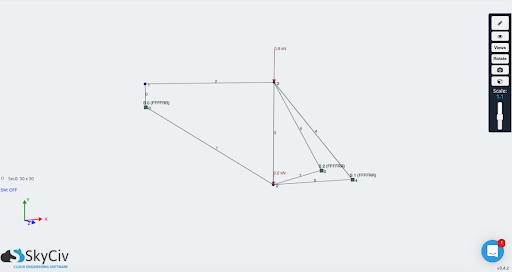2D modeling techniques are commonly used for slab-like elements such as walls, slabs, shells, bridge piers, tanks, silos or domes. For all of these members, the two dimensions perpendicular to the thickness are considered to be much larger than the thickness. It is therefore only the plate thickness that needs to be defined as a section property by the user prior to analysis. However, there are limitations to using 2D modeling techniques as strict rules must be followed to get the most accurate results. The following main rules are the ratio between the thickness and the area of ​​the slab itself, using a slab with a very high ratio of thickness to area will enter the realm of 3D modeling where all the assumptions made with 2D elements no longer exist hold true.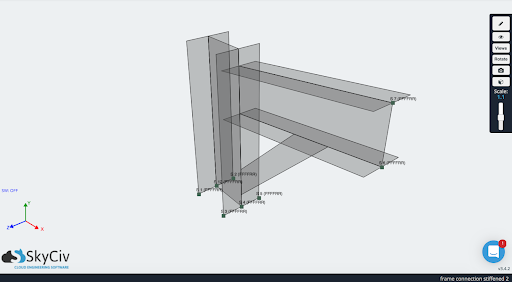3D elements can be used to model any part. In this case, each member of the model is usually divided into equal 3D volumetric elements. As it stands, CAD programs (such as SolidWorks) are used to create these 3D models, it provides the ability to create more complex 3D models for structural analysis. For example, the same bicycle frame as the 1D elements can be replicated using 3D elements, with the following result: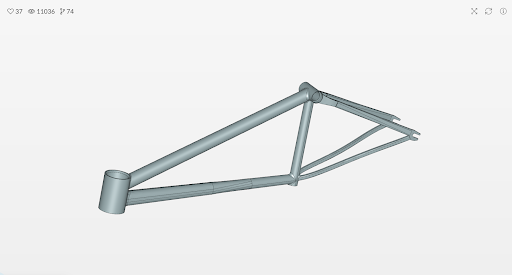Source: SimScale
A major disadvantage of using 3D elements is its modeling (the above model can take up to 10x longer than 1D modeling) and time to solve. 3D elements tries to fully capture the physics of the model, internally and externally, by using the computationally intensive calculations required to solve the analysis at the expense of spending more time on the model itself.

### Networking

Because meshing is a critical step in any structural analysis, it is important that users understand the effects of meshing on 1D, 2D, and 3D elements on a model.

As already mentioned, 1D elements are usually used to represent line elements and can provide precise bending behavior of an element. 1D element meshing is the division of the element into several segments, this does not affect the overall result, but more segments allow smoother and better visualization of the results.

2D and 3D elements have similar characteristics in terms of meshing. Each element within the model is divided into several parts of a specific shape, the mesh size of the model affects the final results and the finer the mesh (smaller shapes used) the longer it takes to solve the model. There are two shapes that are used for both 2D elements in meshing, square and triangular elements. 3D element shapes are variations that result from the 2D element shapes; commonly used shapes are hexahedra, tetrahedron, wedges and pyramids, each with different advantages to better model the physics of the model itself.

### Output (post-processing)

Analysis results for elements modeled using 1D elements are typically reported as the shear force and bending moment about the two major axes of the element, and the axial force and torsional moment about the axis connecting both ends of the element.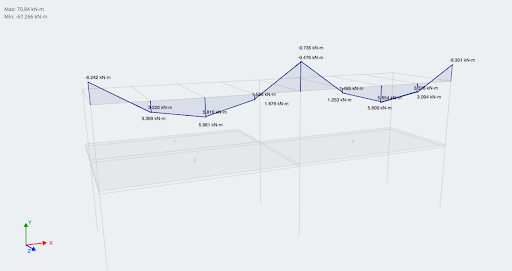Example of a bending moment force diagram in 1D analysis software
For 2D elements, the output is shown as axial force, shear force, bending moment, and torsion moment per unit length.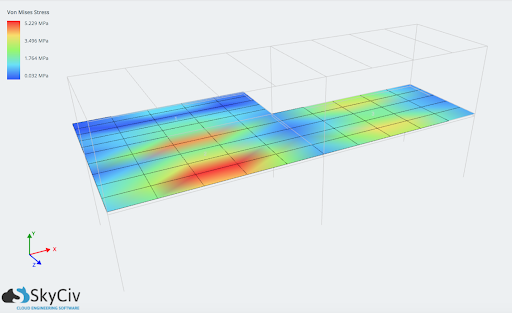Such results can include:

• Membrane forces
• Shear / moment forces in the plane
• Displacements (x, and, with, sum)
• Scheren (Von-mises, Direct, Scheren, main / subsidiary principle)

When, brick elements are adopted, the results are given in terms of stresses. Therefore, the internal forces and support reactions of elements such as shear walls, shells or slabs modeled using 2D elements or brick elements are obtained by integrating internal forces / moments per unit of length or stresses over the length or the area of ​​interest, respectively.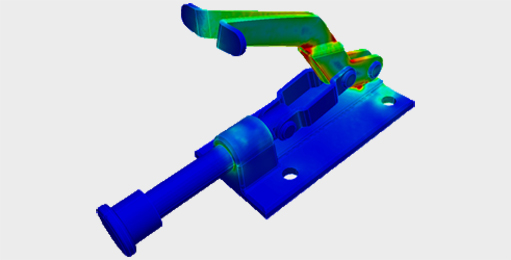Paul Comino
CTO and Co-Founder of SkyCiv
BEng Mechanical (Hons1), BCom